# [KW36] FlexCAN Bit Timing Parameters Setup

cancel
Showing results for
Search instead for
Did you mean:

# [KW36] FlexCAN Bit Timing Parameters Setup

No ratings

## [KW36] FlexCAN Bit Timing Parameters Setup

FRDM-KW36 Software Development Kit (SDK) includes drivers and examples of FlexCAN module for KW36 which can be easily configured for a custom communication. For example, if user want to change the default baud rate from FlexCAN driver demo examples then the only needed change is the default value on "config->baudRate" and "config->baudRateFD" from "FLEXCAN_GetDefaultConfig" function (See Figure 1). Segments within a bit time will be automatically configured to obtain the desired baud rate. By default, demos are configured to work with CAN FD communication.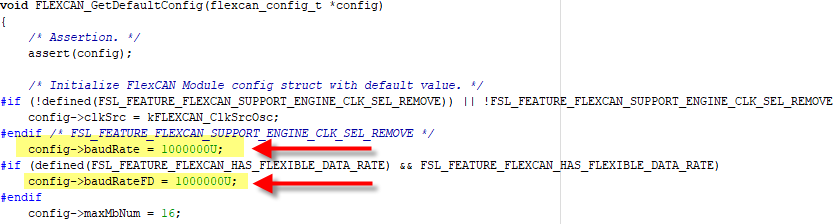Figure 1. FRDM-KW36's default baudrate from flexcan_interrupt_transfer driver example

Even so, there are cases where segments within a bit time are not well configured and it's necessary that user configure segments manually.

An example occurs by setting the maximum FD baud rate "3.2MHz" using the 32MHz xtal or "2.6MHz" using a 26MHz xtal where demo reports an error. See Figure 2.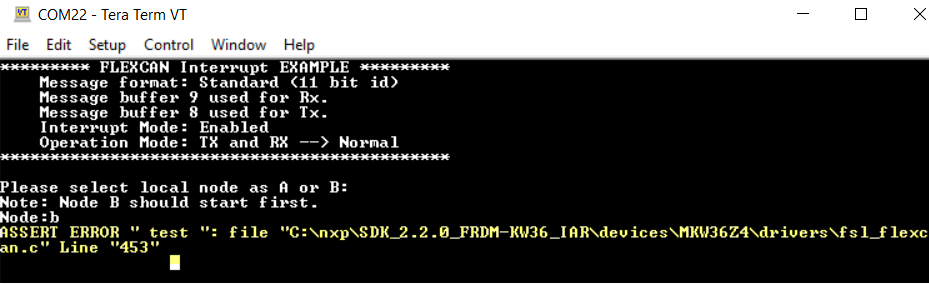Figure 2. Error by setting maximum baud rate

When this error occurs, the fix is on setting the timing config parameters correctly by including the definition of SET_CAN_QUANTUM on application source file (see Figure 3) and then declare and initialize the timing config parameters shown in Figure 4.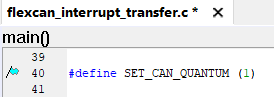Figure 3. SET_CAN_QUANTUM define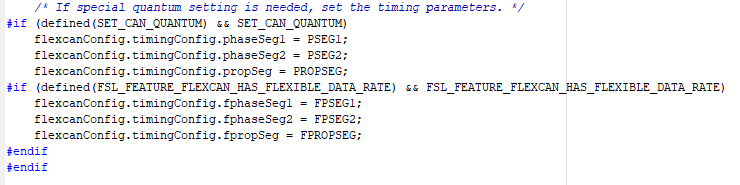Figure 4. Custom timing config parameters

For this example we are going to show how to calculate timing config parameters in an scenario where a CAN FD communication is used with baud rate of 500kHz on nominal phase and 3.2MHz on FD phase. See Figure 5.  To do it, we need to calculate Time Quanta and value of segments within the bit time.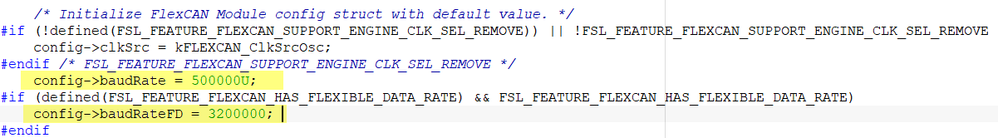Figure 5. Custom CAN FD baudrate

KW36 Reference Manual in chapter "37.4.8.7 Protocol timing" shows the segments within a bit time for CAN nominal phase configured in "CAN_CTRL1" register (see Figure 6), and segments for FD phase configured in CAN_FDCBT register (see Figure 7).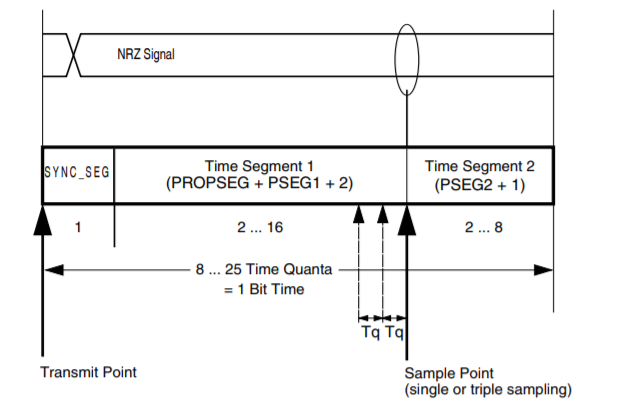Figure 6. Segment within a bit time for CAN nominal phase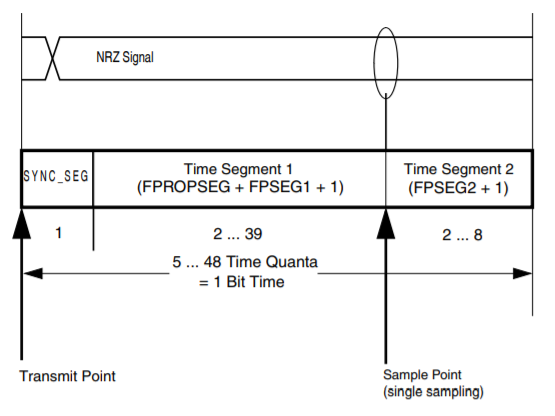Figure 7. Segment within a bit time for CAN FD phase

Before calculating the value of segments, first we need to calculate the Time Quanta which is the atomic number of time handled by the CAN engine.

The formula to calculate Time Quanta is shown in Figure 8 taken from KW36 Reference Manual.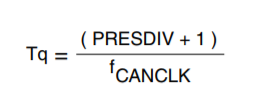Figure 8. Time Quanta Formula

CANCLK can be selected by CLKSRC bits on CAN_CTRL1 register as shown in Figure 9, where the options are Peripheral clock=20MHz or Oscillator clock (16MHz if using 32MHz xtal or 13MHz if using 26MHz xtal). The recomiendation is to use the Oscillator clock due to peripheral clock can have jitter that affect communication.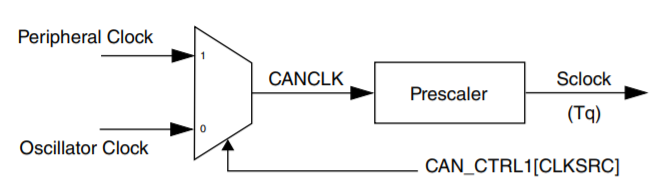Figure 9. CAN clocks

To select the Oscillator clock, search for flexcanConfig.clkSrc definition and set it to kFLEXCAN_ClkSrcOsc as shown in Figure 10.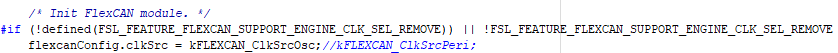Figure 10. CANCLK selection

Next step is selecting the PRESDIV value for nominal phase and FPRESDIV for FD phase. You have to select the right value to achieve the TQ needed to obtain the configured baudrate. For this example, let's set FPRESDIV value to 0 and PRESDIV value to 3.

TQ calculation for nominal phase:

TQ = (PRESDIV + 1) / CANCLK = (3 + 1) / 16000000 = 0.00000025

TQ calculation for FD phase:

TQ = (FPRESDIV + 1) / CANCLK = (0 + 1) / 16000000 = 0.0000000625

The bit rate, which defines the rate of CAN message is given by formula shown in Figure 11 taken from KW36 Reference Manual.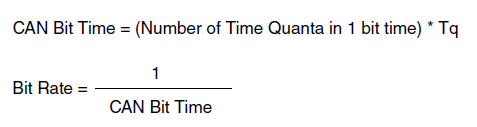Figure 11. CAN Bit Time and Bit Rate Formulas

With this info and with our TQ calculated, we can deduce that we need:

• For Nominal phase: 8 = Number of Time Quanta in 1 bit time
• For FD phase: 5 = Number of Time Quanta in 1 bit time

Now, let's define the value of segments.

For nominal phase:

• Bit Time =  (number of Tq in 1 bit time) x Tq
• CAN Bit Time = (1 + (PROPSEG + PSEG1 + 2) + (PSEG2 + 1) ) x Tq
• CAN Bit Time = (1 + (1 + 2  + 2) + (1 + 1) ) x Tq = 8 x 0.00000025 =
• Baud rate = 1/ CAN Bit Time = 500KHz

For FD phase:

• CAN Bit Time = (number of Tq in 1 bit time) x Tq
• CAN Bit Time = (1 + (FPROPSEG + FPSEG1 + 1) + (FPSEG2 + 1) ) x Tq
• CAN Bit Time = (1 + (0 + 1 + 1) + (1 + 1) ) x Tq = 5 x Tq =  0.0000003125
• Bit Rate = 1/CAN Bit Time = 1 / 0.0000003125 =  3.2MHz

To finish, just update the calculated values on your firmware on flexcanConfig.timingConfig structure.

Notes:

• FRDM-KW36 Software Development Kit (SDK) can be downloaded from MCUXpresso webpage.
• FlexCAN driver examples are located in path: "SDK_2.2.0_FRDM-KW36\boards\frdmkw36\driver_examples" from your downloaded FRDM-KW36 SDK.
• Take in consideration that not all the baud rates are achievables and will depend on the flexcan clock and segment values used.
Labels (2)

• ### KW

Tags (5)
Version history
Revision #:
1 of 1
Last update:
‎10-19-2018 02:19 PM
Updated by: